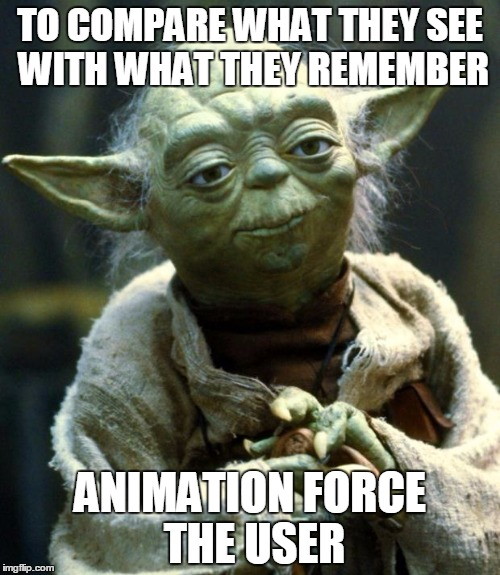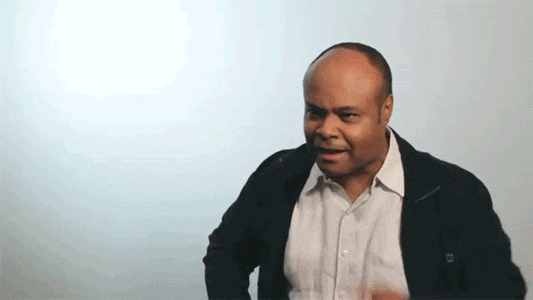# Case Study: Animation and Others Vizs

• June 2016
• R

This post will be about if we can show some data in other ways to try to tell more clearly the Oh! Foo! is this rly happening? story.

Time time ago an gif appears showing the change of the global temperatures over time.Well, some sites like http://gizmodo.com/ made a reference to this animation as one-of-the-most-convincing-climate-change-visualization. Mmmm… ok! A kind of click bait IMHO but at least the title saids visualization :B. But for me the animation don’t work always. I rembember a quote, sadly I don’t rember the author, maybe/surely was Alberto Cairo (If you know it please tell me who was):

Animation force the user to compare what they see with what they remember (saw).

If you want it in Yoda’s way:Other thing I don’t like so much about this spiral is there’are so much data overlaped at the end of animation hiding information about the speed of increment in the data.

## Data & Packages

We’ll use the data provide by hrbrmstr in his repo. Bob Rudis made a beautiful representation of the data via ggplot2 and D3 using a `geom_segment`/column range viz.

About the packages. Here we’ll use a lot of `dplyr`, `tidyr`, `purrr` for the data manipulation, for the colors we’ll use `viridis`, lastly I’ll use `highcharter` for charts

``````library("highcharter")
library("dplyr")
library("tidyr")
library("lubridate")
library("purrr")
library("viridis")

options(
highcharter.theme = hc_theme_darkunica(
chart  = list(
style = list(fontFamily = "Roboto Condensed"),
backgroundColor = "#323331"
),
yAxis = list(
gridLineColor = "#B71C1C",
labels = list(format = "{value} C", useHTML = TRUE)
),
plotOptions = list(series = list(showInLegend = FALSE))
)
)

df <- df %>%
mutate(date = ymd(year_mon),
tmpstmp = datetime_to_timestamp(date),
year = year(date),
month = month(date, label = TRUE),
color_m = colorize(median, viridis(10, option = "B")),
color_m = hex_to_rgba(color_m, 0.65))

dfcolyrs <- df %>%
group_by(year) %>%
summarise(median = median(median)) %>%
ungroup() %>%
mutate(color_y = colorize(median, viridis(10, option = "B")),
color_y = hex_to_rgba(color_y, 0.65)) %>%
select(-median)

df <- left_join(df, dfcolyrs, by = "year")
``````

The data is ready, let’s go.

year_mon median lower upper year decade month date tmpstmp color_m color_y
1850-01-01 -0.702 -1.102 -0.299 1850 1850 Jan 1850-01-01 -3.79e+12 rgba(2,1,10,0.65) rgba(87,16,107,0.65)
1850-02-01 -0.284 -0.675 0.114 1850 1850 Feb 1850-02-01 -3.78e+12 rgba(107,23,108,0.65) rgba(87,16,107,0.65)
1850-03-01 -0.732 -1.080 -0.383 1850 1850 Mar 1850-03-01 -3.78e+12 rgba(1,0,8,0.65) rgba(87,16,107,0.65)
1850-04-01 -0.570 -0.903 -0.237 1850 1850 Apr 1850-04-01 -3.78e+12 rgba(9,4,26,0.65) rgba(87,16,107,0.65)
1850-05-01 -0.325 -0.662 0.006 1850 1850 May 1850-05-01 -3.78e+12 rgba(84,15,107,0.65) rgba(87,16,107,0.65)
1850-06-01 -0.213 -0.515 0.084 1850 1850 Jun 1850-06-01 -3.77e+12 rgba(148,38,100,0.65) rgba(87,16,107,0.65)

## Spiral

First of all let’s try to replicate the chart/gif/animation that’s reason to write this post. Here we’ll construtct a `list` of series to use with `hc_add_series_list` function.

``````lsseries <- df %>%
group_by(year) %>%
do(
data = .\$median,
color = first(.\$color_y)) %>%
mutate(name = year) %>%
list.parse3()

hc1 <- highchart() %>%
hc_chart(polar = TRUE) %>%
hc_plotOptions(
series = list(
marker = list(enabled = FALSE),
animation = TRUE,
pointIntervalUnit = "month")
) %>%
hc_legend(enabled = FALSE) %>%
hc_xAxis(type = "datetime", min = 0, max = 365 * 24 * 36e5,
labels = list(format = "{value:%B}")) %>%
xDateFormat = "%B",
pointFormat = " {series.name}: {point.y}") %>%

hc1
``````

open

Ok! without the animation componet this don’t work so much.

If we want replicate the animation part we can hide all the series using transparency.

``````lsseries2 <- df %>%
group_by(year) %>%
do(
data = .\$median,
color = "transparent",
enableMouseTracking = FALSE,
color2 = first(.\$color_y)) %>%
mutate(name = year) %>%
list.parse3()
``````

Then using a little of javascript we can color each series one by one with the real color.

``````hc11 <- highchart() %>%
hc_chart(polar = TRUE) %>%
hc_plotOptions(series = list(
marker = list(enabled = FALSE),
animation = TRUE,
pointIntervalUnit = "month")) %>%
hc_legend(enabled = FALSE) %>%
hc_title(text = "Animated Spiral") %>%
hc_xAxis(type = "datetime", min = 0, max = 365 * 24 * 36e5,
labels = list(format = "{value:%B}")) %>%
hc_tooltip(headerFormat = "{point.key}", xDateFormat = "%B",
pointFormat = " {series.name}: {point.y}") %>%
hc_chart(
events = list(

function() {
var duration = 16 * 1000
var delta = duration/this.series.length;
var delay = 2000;

this.series.map(function(e){
setTimeout(function() {
e.update({color: e.options.color2, enableMouseTracking: true});
e.chart.setTitle({text: e.name})
}, delay)
delay = delay + delta;
});

}
")
)
)
``````

And voila.

``````hc11
``````

open

You can open the chart in a new window to see the animation effect.

## Sesonalplot

We need polar coords here? I don’t know so let’s back to the euclidean space and see what happened

``````hc2 <- hc1 %>%
hc_chart(polar = FALSE, type = "spline") %>%
hc_xAxis(max = (365 - 1) * 24 * 36e5) %>%
hc_yAxis(tickPositions = c(-1.5, 0, 1.5, 2))

hc2
``````

open

Nom! A nice colored spaghettis. Not so much clear what happened across the years.

## Heatmap

Here we put the years in xAxis and month in yAxis:

``````m <- df %>%
select(year, month, median) %>%
select(-month) %>%
as.matrix()

rownames(m) <- month.abb

hc3 <- hchart(m) %>%
hc_colorAxis(
stops = color_stops(10, viridis(10, option = "B")),
min = -1, max = 1
) %>%
hc_yAxis(
title = list(text = NULL),
tickPositions = FALSE,
labels = list(format = "{value}", useHTML = TRUE)
)

hc3
``````

open

With the color scale used is not that clear the impact about the incremet. We can see the series have and increase but with colors is not so easy to quantify that change.

## Line / Time Series

Let’s try now the most simply chart. And let’s represent the data as a time series.

``````dsts <- df %>%
mutate(name = paste(decade, month)) %>%
select(x = tmpstmp, y = median, name)

hc4 <- highchart() %>%
hc_xAxis(type = "datetime") %>%
hc_yAxis(tickPositions = c(-1.5, 0, 1.5, 2)) %>%
hc_add_series_df(dsts, name = "Global Temperature",
type = "line", color = hex_to_rgba(viridis(10, option = "B")),
lineWidth = 1,
states = list(hover = list(lineWidth = 1)),
hc4
``````

open

maybe it’s so simple. What do you think?

## Columrange

Finally let’s add the information about the confidence interval and add the media information using a color same as hrbrmstr did.

With highcharter it’s easy. Just define the dataframe with `x`, `low`, `high` and `color` and add it to a `highchart` object with the `hc_add_series_df` function.

``````dscr <- df %>%
mutate(name = paste(decade, month)) %>%
select(x = tmpstmp, low = lower, high = upper, name, color = color_m)

hc5 <- highchart() %>%
hc_yAxis(tickPositions = c(-2, 0, 1.5, 2)) %>%
hc_xAxis(type = "datetime") %>%
hc_add_series_df(dscr, name = "Global Temperature",
type = "columnrange")

hc5
``````

open

(IMHO) This is a really way to show what we want to say:

• Via a time series chart it’s wasy compare the past with the actual period of time.
• The color, in particular the last yellowish part, add importance and guide our eyes to that part of the chart before to start to compare.source

Do you have other ways to represent this data?